Test Description

## 25 Questions MCQ Test Mathematics (Maths) Class 10 | Practice Test: Quadratic Equations

Practice Test: Quadratic Equations for Class 10 2023 is part of Mathematics (Maths) Class 10 preparation. The Practice Test: Quadratic Equations questions and answers have been prepared according to the Class 10 exam syllabus.The Practice Test: Quadratic Equations MCQs are made for Class 10 2023 Exam. Find important definitions, questions, notes, meanings, examples, exercises, MCQs and online tests for Practice Test: Quadratic Equations below.
Solutions of Practice Test: Quadratic Equations questions in English are available as part of our Mathematics (Maths) Class 10 for Class 10 & Practice Test: Quadratic Equations solutions in Hindi for Mathematics (Maths) Class 10 course. Download more important topics, notes, lectures and mock test series for Class 10 Exam by signing up for free. Attempt Practice Test: Quadratic Equations | 25 questions in 25 minutes | Mock test for Class 10 preparation | Free important questions MCQ to study Mathematics (Maths) Class 10 for Class 10 Exam | Download free PDF with solutions
 1 Crore+ students have signed up on EduRev. Have you?
Practice Test: Quadratic Equations - Question 1

### Which of the following quadratic expression can be expressed as a product of real linear factors?

Detailed Solution for Practice Test: Quadratic Equations - Question 1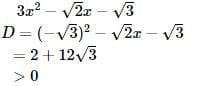Thus, it can be expressed as product of linear factors.

Practice Test: Quadratic Equations - Question 2

### Two candidates attempt to solve a quadratic equation of the form x2 + px + q = 0. One starts with a wrong value of p and finds the roots to be 2 and 6. The other starts with a wrong value of q and finds the roots to be 2 and – 9. Find the correct roots of the equation :

Practice Test: Quadratic Equations - Question 3

### Solve for x : 15x2 – 7x – 36 = 0

Detailed Solution for Practice Test: Quadratic Equations - Question 3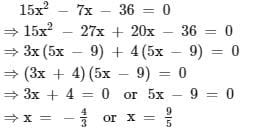Practice Test: Quadratic Equations - Question 4

Solve for y : (√7) y2 – 6y –13 (√7) = 0

Detailed Solution for Practice Test: Quadratic Equations - Question 4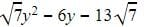Using mid term splitting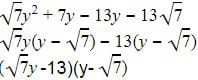So either y=13/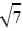Or y=Practice Test: Quadratic Equations - Question 5

Solve for x : 6x2 + 40 = 31x

Practice Test: Quadratic Equations - Question 6

Determine k such that the quadratic equation x2 + 7(3 + 2k) – 2x (1 + 3k) = 0 has equal roots :

Practice Test: Quadratic Equations - Question 7

Discriminant of the equation – 3x2 + 2x – 8 = 0 is

Detailed Solution for Practice Test: Quadratic Equations - Question 7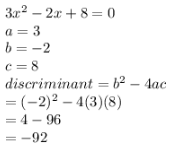Practice Test: Quadratic Equations - Question 8

The nature of the roots of the equation x2 – 5x + 7 = 0 is –

Detailed Solution for Practice Test: Quadratic Equations - Question 8

Given equation is x2-5x+7=0
We have discriminant as b2-4ac=(-5)2-4*1*7= -3
And x =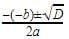, Since we do not have any real number which is a root of a negative number, the roots are not real.

Practice Test: Quadratic Equations - Question 9

The roots of a2x2 + abx = b2, a = 0 are :

Practice Test: Quadratic Equations - Question 10

The equation x2 – px + q = 0 p, q ε R has no real roots if :

Practice Test: Quadratic Equations - Question 11

Determine the value of k for which the quadratic equation 4x2 – 3kx + 1 = 0 has equal roots :

Practice Test: Quadratic Equations - Question 12

Find the value of k such that the sum of the squares of the roots of the quadratic equation x2 – 8x + k = 0 is 40:

Practice Test: Quadratic Equations - Question 13

Find the value of p for which the quadratic equation x2 + p(4x + p – 1) + 2 = 0 has equal roots :

Practice Test: Quadratic Equations - Question 14

The length of a hypotenuse of a right triangle exceeds the length of its base by 2 cm and exceeds twice the length of the altitude by 1 cm. Find the length of each side of the triangle (in cm) :

Practice Test: Quadratic Equations - Question 15

A two digit number is such that the product of it's digits is 12. When 9 is added to the number, the digits interchange their places, find the number :

Practice Test: Quadratic Equations - Question 16

A plane left 40 minutes late due to bad weather and in order to reach it's destination, 1600 km away in time,it had to increase it's speed by 400 km/h from it's usual speed. Find the usual speed of the plane :

Practice Test: Quadratic Equations - Question 17

The sum of the squares of two consecutive positive odd numbers is 290. Find the sum of the numbers :

Practice Test: Quadratic Equations - Question 18

A shopkeeper buys a number of books for Rs. 80. If he had bought 4 more for the same amount, each book would have cost Re. 1 less. How many books did he buy?

Practice Test: Quadratic Equations - Question 19

Two squares have sides x cm and (x + 4) cm. The sum of their areas is 656 cm2. Find the sides of the square.

Practice Test: Quadratic Equations - Question 20

The real values of a for which the quadratic equation 2x2 – (a3 + 8a – 1) x + a2 – 4a = 0 possesses roots of opposite signs are given by :

Practice Test: Quadratic Equations - Question 21

The number of real solutions of the equation is :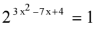Practice Test: Quadratic Equations - Question 22

If the equation (3x)2 + (27 × 31/k  – 15) x + 4 = 0 has equal roots, then k =

Practice Test: Quadratic Equations - Question 23

If x =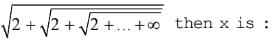Practice Test: Quadratic Equations - Question 24

Equation ax2 + 2x + 1 has one double root if :

Practice Test: Quadratic Equations - Question 25

Solve for x : (x + 2) (x – 5) (x – 6) (x + 1) = 144 :

## Mathematics (Maths) Class 10

25 videos|370 docs|138 tests(Scan QR code)